PHYSICS Mass MASS MA. Fundamental of Mass MB. Kong Frequency and Kong Wavelength MC. Annihilation and Pair Production MD. Kong Equation ME. Kong Atom Model MF. Quantum of Atom MG. Perturbation of Photon MH. Periodic Table MI. Chemical Reaction MJ. Superconductor MK. Particles and Waves ML. Nuclear Physics MC. ANNIHILATION AND PAIR PRODUCTION 1. Annihilation 2. Pair Production 3. Principles of Conservations 5. Intrinsic Spin Angular Momentum of Electron 6. Spin Magnetic Dipole Moment of Electron INTRODUCTION   From the chapter "Fundamental of Mass”, it is quoted that wave is defined as kinetic-time-energy and mass is defined as potential-time-energy. Wave travels at the speed of light, the possible fastest speed. It is a moving energy; therefore it is a kinetic energy. Wave has no time property; it is also defined as kinetic-time-energy at time zero. Mass is a converse of wave. The converse of the kinetic-time-energy is the potential-time-energy.   Annihilation and pair production are two processes involve the transformation between wave and particles. Annihilation is the transformation from particles to waves or the conversion of the potential-time-energy to the kinetic-time-energy. Pair production is the transformation from waves to particles, or the conversion of the kinetic-time-energy to the potential-time-energy.   The annihilation process gives a very good hint to the nuclear physics and the pair production process gives a very good hint to the ingredients of mass. From these two processes, we can observe clearly on the ingredients of the mass and wave which are able to interchange.   This chapter also derives the radius of electron and the half spin of the electron from the pair production process.     OBJECTIVES   1)      To explain, postulate and illustrate the annihilation and pair production process. 2)      To develop the Kong radius, which is the radius of electron. 3)      To derive the spin angular momentum of electron. 4)      To derive the spin magnetic moment of electron. MC.1.0        ANNIHILATION   Annihilation process happens on 2 particles which have exactly the opposite M&E characteristic, such as electron and positron. When both particles are attracted and combined together, the M&E field is restructured and released to become light or photon. The annihilation process is illustrated as follows, e- + e+ à γ                                                                                          … eq. MC.1.1   For annihilation, the charge for the system is conserved. The electron, e- whose possesses negative charge and the positron, e+ whose is positive charge, both undergo annihilation process and transform into gamma rays, whose is chargeless. As described in eq. MC.1.1, the net charge of the system at both sides is zero.   In annihilation, two particles structure are combined and restructured to become the wave structure. Figure MC.1.1 below postulate and illustrate the stages of the transformation from particles to photon.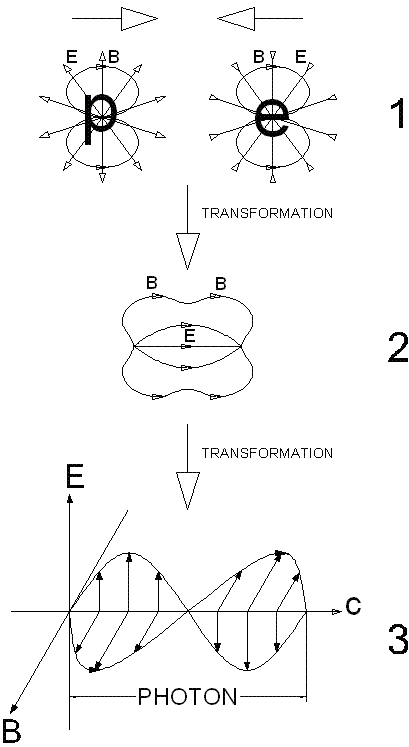Figure MC.1.1   From figure MC.1.1, stage 1 shows the structure of the positron (p) and electron (e). Both have the magnetic (B) and electric (E) (M&E) field as the nucleus. The positron and electron attract to each other due to the electrostatic and magnetic force and transform to stage 2.   Stage 2 shows the combination of the annihilation process. The combination causes the change in M&E fields. According to the Faraday’s law and the Maxwell’s law of induction, the change of magnetic field produces electric field and the change of electric field produces magnetic field. The inter-induction of M&E field then transforms to the photon as shown in stage 3 of figure MC.1.1.   The dot product in the Faraday’s and Maxwell’s law of induction between the change of M&E flux and the M&E field produced is always perpendicular. Therefore, the induced photon always travels at the direction perpendicular to the change of M&E flux.   In this annihilation process, the combination process also implies the nuclear reaction process where the interaction of the nucleus of particles takes place. It is also observed that the photon and particle have the same ingredients, which are the M&E field.     MC.1.1        Conservation of Energy for Annihilation   The Grand Energy Equation, E2 = (pc)2 + (mc2)2                                                                              … eq. MC.1.2 where   c = speed of light   The first term of right side, pc, refers to electromagnetic wave, which is the kinetic-time-energy. While the second term of right side, mc2, refers to the mass, which is the potential-time-energy.   The conservation of energy for the system is, E12 = E22                                                                                             … eq. MC.1.3   E12 = (mpc2 + mec2)2                                                                          … eq. MC.1.4 E22 = (pg,oc)2 = (hfg,o)2                                                                       … eq. MC.1.5 where   h = Plank’s constant             fg,o = incident frequency of gamma photon             pg,o = momentum of gamma photon at incident frequency             me = mp = mass of electron and positron             c = speed of light   Equating eq. MC.1.4 and eq. MC.1.5, we obtain, 2mec2 = hfg,o                                                                                        … eq. MC.1.6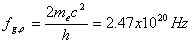… eq. MC.1.7   Eq. MC.1.7 is the frequency of the gamma photon produced by annihilation process.     MC.1.2        Conservation of Momentum for Annihilation   From figure MC.1.1, the linear momentum at x-direction of the system is mpvx – mevx = pe,x – pp,x = 0                                                                 … eq. MC.1.8 The linear momentum of the particle system is conserved.   The angular momentum of the system is expressed as follow Se – Sp = 0                                                                                              … eq. MC.1.9 The spin angular momentum of the particle system is conserved.   In annihilation process, the particle is converted to photon, the momentum of the conversion happens at perpendicular angle. The momentum of the photon is the summation of the particles in magnitude. The conservation of momentum between the particles and photon is expressed as follow pg,o = pe + pp = 2pe                                                                             … eq. MC.1.10 MC.2.0        PAIR PRODUCTION   Pair production is the converse of the annihilation. Gamma photon is transformed into two particles. To differentiate the two particles, they are named electron and positron.   Figure MC.2.1 shows the pair production event that occurred in a bubble chamber. A gamma photon enters into the chamber from the left. The merged point in figure MC.2.1 is the point where the pair production happened. Due to the charged property, the electron and positron left a trail of tiny bubbles moving from left to right.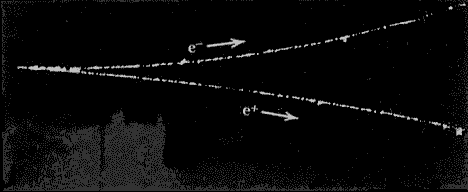Figure MC.2.1   The pair production process is illustrated as follow, γ à e- + e+                                                                                          … eq. MC.2.1   For pair production to happen, Gamma photon must have the minimum frequency of fg,o = 2.47x1020 Hz                                                                               … eq. MC.2.2   or maximum wavelength of lg,o = 1.21x10-12 m                                                                               … eq. MC.2.3   For gamma photon that possesses higher frequency than the minimum value, the excess energy is converted to kinetic energy of produced particles.   From the chapter “Photon”, the size of photon is quoted as follow,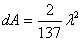… eq. MC.2.4   Hence, the size of gamma photon is, dA = 2.14x10-26 m2                                                                              … eq. MC.2.5   From the chapter “Kong Atom Model” of hydrogen, the radius of hydrogen atom is the Bohr radius. rB = 5.29x10-11 m2                                                                                … eq. MC.2.6   Hence, the cross sectional area of atom hydrogen is, AH = prB2 = 8.79x10-21 m2                                                                   … eq. MC.2.7   From eq. MC.2.5 and eq. MC.2.7, the size of gamma photon is much smaller than the cross sectional area of the atom hydrogen and smaller than the outward portion of the first magnetic gauss line of the atom hydrogen. Therefore, gamma photon is able to enter into the center of hydrogen atom. This is how and where the pair production takes place.   The pair production process is suggested and illustrated in figure MC.2.2. As described in the Kong atom model, the center (origin) of atom is the point where electric and magnetic field are merged.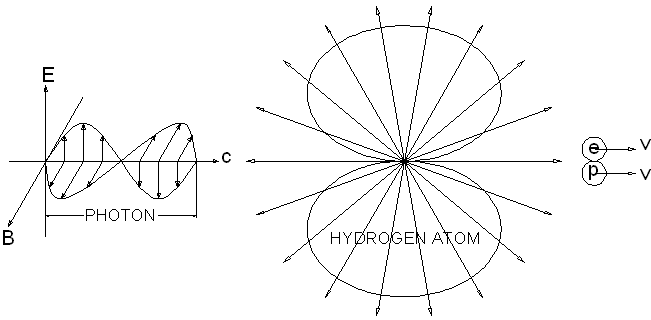Figure MC.2.2   When gamma photon reaches the origin point of atom hydrogen, the two perpendicular components, which are the magnetic and electric (M&E) components, of gamma photon deforms into a point.   When the two M&E vector components of photon are reduced and merged into a point, they deform into two separate M&E component with a common origin, which are the M&E field of charged particles. The structure of charged particle is similar to the atom model where the electric field is distributed spherically from an origin point and the magnetic field is an inner merged toroid. All mass possess the same structure model.     MC.2.1        Transformation of Pair Production   From the topic ‘Photon’, photon is described as the energy per unit of wavelength. For one unit of wavelength or photon, the M&E profile is described by one complete sinusoidal profile. One complete sinusoidal profile of M&E field has two peaks and one merged neutral in between. One peak is described and defined as positive and another one is negative. The first peak or the first half of photon produces one particle and the second half of photon produce another particle. These two particles are differentiated with the name electron and positron.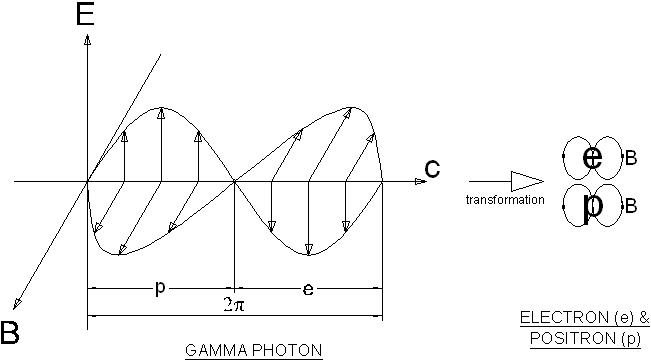Figure MC.2.3   Figure MC.2.3 suggests the transformation of gamma photon to electrons. The M&E field of photon is transformed to become particles. The magnetic field is transformed to become an inner merged toroid, while the electric field is transformed and become a point charge.  The detail transformation is postulated in figure MC.2.4 below.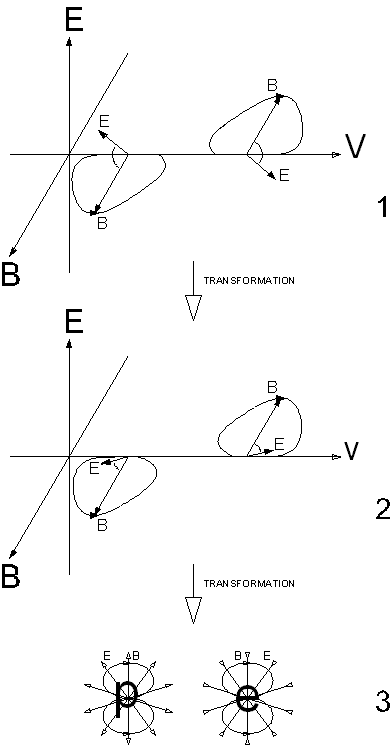Figure MC.2.4   Stage 1 of figure MC.2.4 is the change of the Kong vector. (Refer to the topic Photon for more descriptions on the Kong vector.) When the gamma photon enters into the M&E field of the atom hydrogen before reaches the origin point, the M&E field of gamma photon is twisted or deformed. The deform M&E field of the photon reduces the velocity of photon from the speed of light.   Stage 2 shows that when the photon enters deeper into the center of the atom hydrogen, the deformed angle increases and the velocity of the photon become slower and almost stagnant.   When the gamma photon reaches the center of the atom hydrogen, the electric field of the photon is fully squeezed into a point and become a point charge where the deformed electric field becomes spherical. The squeezed photon then becomes the charge particles as shown in stage 3 of figure MC.2.4.   The charge particles are separated perpendicular to the direction of the incident photon and the electron and positron travel in opposite direction.   Pair production is a process where the kinetic-time-energy is converted to the potential-time-energy. For a gamma photon, the photon energy can be expressed as,   E = pg,oc = hfg,o                                                                                   … eq. MC.2.8 where   pg,o = momentum of gamma photon at incident frequency             c = speed of light h = plank constant             fg,o = frequency of photon at incident frequency   Rearrange eq. MC.2.8, we obtain the momentum of photon,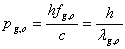… eq. MC.2.9 The gamma photon is divided into two equal parts, the positive and negative part. Positive part produces positron and negative part produces electron.   Half of the gamma photon possesses half of the momentum as described below, pg,o = pe + pp = 2pe                                                                              … eq. MC.2.10 where   pg,o = incident momentum of gamma photon             pe = pp = momentum of electron or positron   Eq. MC.2.10 is equivalent to eq. MC.1.10, the momentum of the system is conserved. More descriptions on the energy and momentum of photon are discussed in the topic photon. MC.3.0        PRINCIPLES OF CONSERVATIONS   Pair Production adhere to the principles of conservations, which includes the following, 1) Conservation of Energy 2) Conservation of Linear Momentum 3) Conservation of Angular Momentum 4) Conservation of Charge   The above 4 principles are discussed below.     MC.3.1        Conservation of Energy   The Grand Energy Equation, E2 = (pc)2 + (mc2)2                                                                              … eq. MC.3.1 where   c = speed of light     MC.3.1.1        At Incident Energy   For pair production, at incident energy, the conservation of energy of the system is, E12 = E22                                                                                             … eq. MC.3.2   Left side of eq. MC.3.2 is the photon energy while the right side is the mass energy as follows,  E12 = (pg,oc)2                                                                                        … eq. MC.3.3 E22 = (mpc2 + mec2)2                                                                           … eq. MC.3.4 where   pg,o = momentum of gamma photon at incident energy             me = mp = mass of electron and positron             c = speed of light   Equating eq. MC.3.3 and eq. MC.3.4, we obtain pg,oc = (mpc2 + mec2) = 2mec2                                                             … eq. MC.3.5   Substitute eq. MC.2.8 into eq. MC.3.5, we obtain hfg,o = 2mec2                                                                                          ... eq. MC.3.6   Rearrange eq. MC.3.5, we obtain pg,o = mpc + mec = 2mec                                                                      … eq. MC.3.7   Substitute eq. MC.2.10 into eq. MC.3.7, we obtain the momentum of the electron is pe = mec                                                                                                 … eq. MC.3.8     MC.3.1.2        Higher Photon Energy   For gamma photon at higher frequency than incident frequency, the photon momentum is, E1 = pgc                                                                                               … eq. MC.3.9 E2 = 2(mec2) + 2K = 2(mec2) + 2(g - 1)mec2 = 2gmec2                     … eq. MC.3.10 where   K = Kinetic energy             g = Lorentz factor   Equating eq. MC.3.9 and eq. MC.3.10, we obtain the momentum of gamma photon, pgc = 2gmec2                                                                                        … eq. MC.3.11   Subtract eq. MC.3.5 with eq. MC.3.11, the kinetic energy of electron and positron after incident is, 2K = 2(g - 1)mec2 =  (pg – pg,o)c                                                         … eq. MC.3.12     MC.3.2        Conservation of Linear Momentum   The conservation of linear momentum of pair production is divided into two parts. From eq. MC.2.10, it is quoted below, pg,o = pe + pp = 2pe                                                                              … eq. MC.2.10 where   pg,o = incident momentum of gamma photon             pe = pp = momentum of electron and positron   The incident momentum of gamma photon is equivalent to the momentum of electron and positron in magnitude. The electron and positron travel at opposite direction.   For gamma photon at higher frequency than incident frequency, the excess of momentum is converted to the linear momentum of electron and positron after incident.   From figure MC.2.1, the initial and final for linear momentum of the system is as follows,   At horizontal direction, pg,x – pg,o = mpvx,p + mevx,e                                                                … eq. MC.3.13   At vertical direction, 0 = mpvy,e + mevy,e                                                                               … eq. MC.3.14      MC.3.3        Conservation of Angular Momentum   The conservation of angular momentum for pair production is expressed as follows, L1 = L2                                                                                                … eq. MC.3.15 where   L1 = initial angular momentum before incident             L2= resultant angular momentum after incident   From figure MC.2.1, the initial and final angular momentum of the system is as follows,  L1 = 0                                                                                                  … eq. MC.3.16 L2 = Se + Sp                                                                                        … eq. MC.3.17 where   Se = intrinsic angular momentum of electron             Sp = intrinsic angular momentum of positron   Equating eq. MC.3.16 and MC.3.17, we obtain, Se = -Sp                                                                                                … eq. MC.3.18   Eq. MC.3.18 tells the intrinsic spin angular momentum for electron and positron. The angular momentum of the electron and positron is at opposite direction. The angular momentum of pair production is conserved.     MC.3.4        Conservation of Charge   Referring to eq. MC.2.1, the pair production process is quoted as follow, γ à e- + e+                                                                                          … eq. MC.2.1   The photon whose is chargeless, produces electron, e- whose possesses negative charge and positron, e+ whose is positive charge. The net charge of the system at both sides is zero. MC.4.0        KONG RADIUS – RADIUS OF ELECTRON   In the pair production process, the energy of the system remains unchanged. The electron or positron spins at the velocity of the speed of light. c = rewe                                                                                               … eq. MC.4.1 where   re = radius of electron             we = angular frequency of electron   Refer to figure MC.2.3 and MC.2.4, the frequency of the system remains unchanged. The electron and positron possess the same angular frequency as the frequency of gamma photon. we = 2pfg,o                                                                                           .. eq. MC.4.2   Substitute eq. MC.3.6 into eq. MC.4.2, we obtain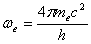… eq. MC.4.3   Substitute eq. MC.4.3 into eq. MC.4.1 and rearrange, we obtain the radius of electron,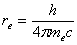… eq. MC.4.4   rK = re = 1.93 x 10-13 m                                                                      … eq. MC.4.5   rK is the Kong radius, the radius of electron. MC.5.0        INTRINSIC SPIN ANGULAR MOMENTUM OF ELECTRON   The basic angular momentum equation for mass is, L = mvr = pr                                                                                         … eq. MC.5.1 where   r = angular radius   The angular momentum of electron is named the spin angular momentum of electron. It is given as follow, S = mevre = pere                                                                                   … eq. MC.5.2 where   me = mass of electron             pe = mev = momentum of electron             re = radius of electron   From eq. MC.3.8, we quote, pe = mec                                                                                               … eq. MC.3.8   Substitute eq. MC.3.8 and eq. MC.4.4 into eq. MC.5.2, the intrinsic spin angular momentum of electron is,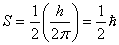… eq. MC.5.3 where= reduced Plank's constant     MC.5.1        Energy Approach   The total energy of the stagnant electron is the potential energy of the electron. The potential energy of mass is equivalent to the intrinsic angular energy of the electron. The intrinsic angular energy of electron is   E = mec2 = Iewe2                                                                                  … eq. MC.5.4   where   me = mass of electron             c = speed of light             mc2 = U = potential energy of mass             Ie = Rotational Inertia of electron             we = angular frequency of electron   The spin angular momentum of electron can be expressed as follow, S = Iewe                                                                                               ... eq. MC.5.5   Substitute eq. MC.5.5 into eq. MC.5.4, we obtain mec2 = Swe                                                                                           … eq. MC.5.6   Substitute eq. MC.4.3 into eq. MC.5.6 and rearrange, we obtain the spin angular momentum,… eq. MC.5.7   Rearrange eq. MC.5.5, we obtain the rotational inertia of electron, Ie = mere2                                                                                            … eq. MC.5.8   The Intrinsic Spin angular momentum of electron is equivalent to the half of the first quantum angular momentum of electron in atom. Two electrons circulate in the same direction in the atom to make a full spin of the orbital of atom. Refer to the chapter “Kong Equation” for more descriptions.   A ± sign is assigned to the intrinsic spin angular momentum to differentiate between electron and positron for counter-clockwise and clockwise spin direction. The intrinsic spin angular momentum is,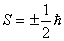… eq. MC.5.9   From the above derivations and explanations, the pair production shows the transformation from photon to particles or mass. The photons and mass both possess the same ingredients, which is the M&E field.   The kinetic-time-energy of photon is converted into the potential-time-energy of mass. The potential energy of mass is the spinning energy of M&E field. The spinning of M&E field produces mass. The spinning M&E field produces the spin angular momentum and the magnetic dipole moment of particles.   All mass possess the same M&E structure. It is predicted that electron is the smallest mass because it spins at the rate of speed of light. MC.6.0        SPIN MAGNETIC DIPOLE MOMENT OF ELECTRON   Pair production is the process of transformation from kinetic-time-energy to potential-time-energy. To convert from the kinetic energy to potential energy, work is required. From the topic “Wave and Photon”, a diagrammatic photon is quoted and shown in figure MC.6.1. The structure of the photon is discussed in detail in the chapter “Kong Vector”. MC.6.1 suggests the transformation process. One half of the photon transforms to electron and another half of the photon transform to positron. m is the magnetic dipole moment of the electron and positron.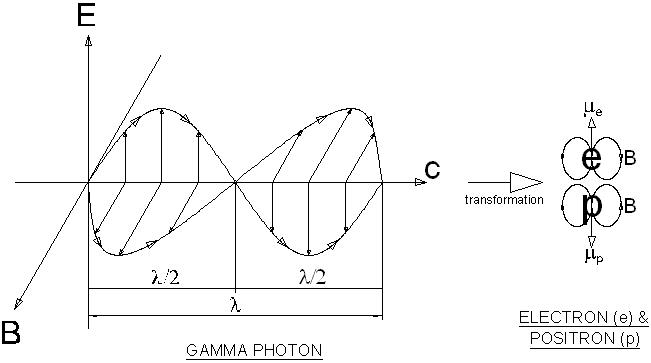Figure MC.6.1   From the chapter “Photon”, the magnetic field of photon is derived as follow,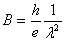… eq. MC.6.1 where   h = Plank’s constant             e = electron charge             l = wavelength of photon   From figure MC.6.1, the wavelength of the electron is le = lg,o/2                                                                                            … eq. MC.6.2   Substitute eq. MC.6.2 into eq. MC.6.1, we obtain the magnetic field of the electron,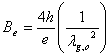… eq. MC.6.3   From eq. MC.3.6 as quoted below, hfg,o = 2mec2                                                                                        ... eq. MC.3.6   The wavelength of gamma photon is,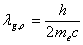… eq. MC.6.4 where   c = fg,olg,o   Substitute the gamma wavelength of eq. MC.6.4 into eq. MC.6.3, we obtain… eq. MC.6.5 The magnetic field of electron is given in eq. MC.6.5.   From the chapter “Energy of Photon”, work is done to squeeze the photon to become the particles. In squeezing the photon, the magnetic and electric dipoles are deformed to a point. The work done is expressed as follow, W = tf                                                                                                  … eq. MC.6.6 where   τ = torque, angular force between electric and magnetic component             f = twisting or deform angle of M&E components   By squeezing the M&E component of photon at the angle of p/2, the work done is equivalent to the potential energy of the electron and positron, W =2mec2                                                                                            … eq. MC.6.7   And the deformed angle is f = p/2                                                                                                … eq. MC.6.8   Substitute eq. MC.6.7 and eq. MC.6.8 into eq. MC.6.6, we obtain the torque as follow… eq. MC.6.9   The squeezing of M&E field of photon to particles produces the magnetic dipole characteristic of particles. The torque required to squeeze the photon can be expressed in the vector relation of magnetic dipole moment and magnetic field as follow, t = me ´ Be                                                                                          … eq. MC.6.10 where   me = magnetic dipole moment of the electron             Be = magnetic field of the electron   Figure MC.6.2 shows the vector relations for the torque, the magnetic field and the magnetic dipole moment. When the M&E components of the photon are deformed by the torque, the magnetic dipole moment is produced. The vector of the magnetic dipole moment is perpendicular to the vector of magnetic field and torque.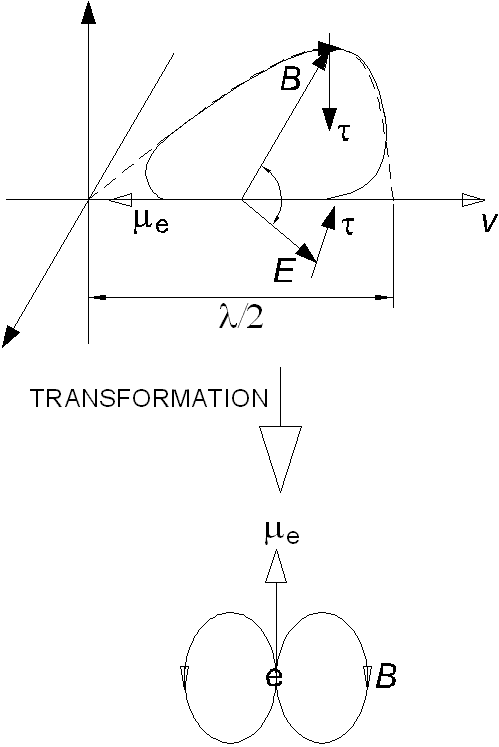Figure MC.6.2   Hence, eq. MC.6.10 becomes, t = meBe                                                                                               … eq. MC.6.11   Substitute eq. MC.6.5 and eq. MC.6.9 into eq. MC.6.11, rearrange to obtain the magnetic dipole moment,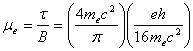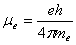… eq. MC.6.12   me = 2msmB                                                                                         … eq. MC.6.13 where   ms = ½ = Spin angular momentum of electron= Bohr magneton   Eq. MC.6.12 is the spin magnetic dipole moment of electron, which has the value similar to the Bohr magneton. DISCUSSIONS AND CONCLUSIONS   Annihilation and pair production are two processes that involve the transformation between particles and photon or the conversion between the potential-time-energy and the kinetic-time-energy respectively. Both processes adhere to the principles of conservations.   The transformation shows the changes of the M&E structure of the photon and particles. It also shows that the M&E fields are the ingredients for the photon and particles. The annihilation process also implies the processes of the nuclear reactions.   The radius of electron is the Kong radius which has the value of rK = lg,o / 2p = 1.93x10-13 m.   The perpendicular sinusoidal structure of the M&E field of photon is transformed to the spinning M&E field of particles. The spin angular momentum of electron is half of the reduced Plank’s constant. Electron possesses half spin.   The spin magnetic dipole moment of electron is same as the Bohr magneton. Spin angular momentum is twice as effective as orbital angular momentum in generating magnetism.

This website is originated on 15-Mar-2007,

updated on 4-Jan-2009.